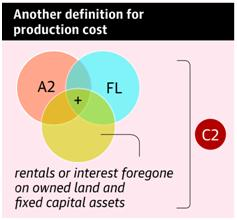# Agriculture Cost of Production

• Posted By
10Pointer
• Categories
Economy
• Published
26th Dec, 2020

## Context

The farmers across India are protesting and one of main reason behind the farmers' protest is the Minimum Support Price (MSP) for crops which raises question on the cost of Production.

How the cost of agricultural produce is calculated?

• Fixed costs: - These are fixed. In agriculture, land in some sense is a fixed capital. The other important items of fixed costs are implements and tools, machinery, farm buildings, work animals etc.
• Variable costs: - These costs vary with the production. One can increase or decrease their use. In agriculture, cost of seed, manure’s and fertilizers, irrigation, labour are the variable costs.The sum of fixed costs and variable costs forms the ‘total cost’, when the total expenditure is deducted from the total returns (income), one gets the ‘net profit’.

## How the different costs are categorized?

• Cost –A: Actual paid out costs for owner cultivator. This cost approximates the actual expenditure incurred in cash and kind and includes the following items:
• Hired human labour
• Owned and hired bullock labour
• Seeds
• Manures and fertilisers
• Implement charges
• Land revenew and other taxes
• Irrigation charges
• Other miscellaneous charges
• Cost –A-1: Corresponding cost for the tenant cultivator, i.e. including rent actually paid by him.
• Cost –A-2: Cost A plus imputed value of own labour.
• Cost – B: Cost A plus rental value of owned land and imputed interest on demand capital.
• Cost – C: total of all cost items, actual as well as imputed.

## How is MSP Calculated?

 The Swaminathan Committee prescribed three variables to determine the production cost. These three variables are: A2, A2+FL and C2. As per the Committee, the ideal formula to calculate the MSP would be: MSP  = C2+ 50% of C2

The ‘Price Policy for Kharif Crops: The Marketing Season 2018-19’ of the CACP stated the given 1.5 times formula to calculate the MSP:

• 5 times MSP Formula = 1.5 times the A2+FL costs